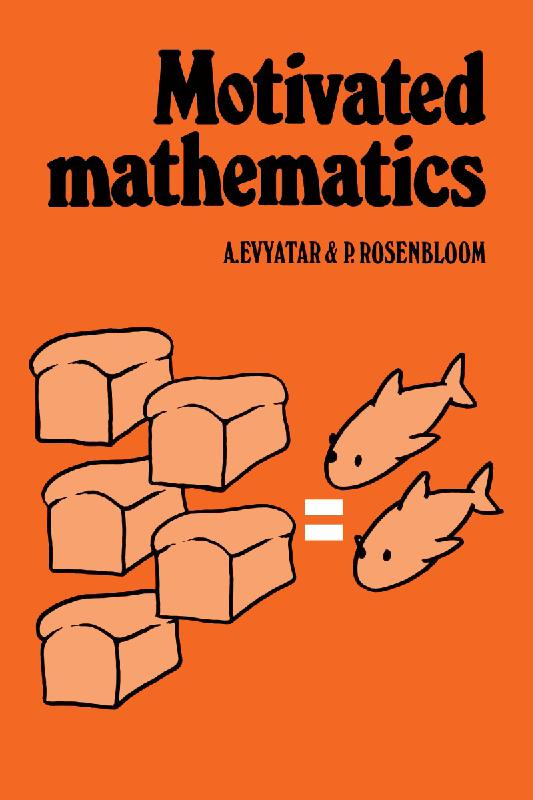### Book Toankho

Sách hay - Sách đẹp

# Motivated MathematicsThe ideas of mathematics can be understood through the techniques needed to solve problems which crop up in everyday life. Conversely, these problems can illustrate how mathematics develops ‘naturally’. The essence of this informal book is to motivate mathematics by examining mathematical models of situations and problems that occur in the real world. Each chapter deals with a specific mathematical topic and each topic is introduced at different levels to provide motivation for students of varying mathematical maturity. The authors emphasize that although applications provide motivation, they are not trying to supply aids for teaching applied mathematics. It is mathematical notions and how they can be developed that are important. We thus have an interesting inversion of aims: physics, biology, linguistics, etc., are here applied to the teaching of mathematics. The bank of ideas in this practical manual will be valuable to teachers of mathematics at schools, colleges and, to a certain extent, universities. Student teachers will also find here a great deal of material of use and interest.